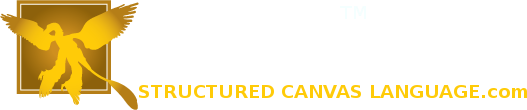# Chaining alts together to transition effects in SCL

Sprite effects in SCL™ are called Alterations because they alter the sprite in some way. When an alteration completes an event can be fired for which you can instruct SCL™ to do something else. That something else can be either to run a routine or to apply one or more alterations. Being able to apply a new alteration when an alteration completes allows for making a chain of alterations.
Anonymous alterations are alterations that are not given a name but are defined within the completion event.
Example:
create sprite from 2.png as whatever
having alt=(sub // use 'sub' to embed definition. The alteration is unnamed.
create slide
where direction=0 distance=50
end)
end

In the example below (which you can run in the develop studio scratchpad) a sprite is moved around using a chain of alts. The first alteration requires a name, FirstSlide, so that the last alteration can refer back it to restart the sequence.
create sprite from 2.png as whatever
having alt=(sub
create slide as FirstSlide // named so we can re-call it
where direction=0 distance=50 completion=(sub
create slide
where direction=270 distance=50 completion=(sub
create rotation
where speed=600 distance=720 completion=(sub
create translation
where x=0 y=0 completion=FirstSlide
end) end) end) end)
end
create routine as Start launch whatever end